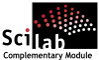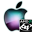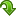ATOMS : Linear Algebra details

# Linear Algebra

A collection of algorithms for linear algebraDetails
Version
0.3.1
A more recent valid version exists: 0.4
Authors
Michael Baudin
Sylvan Brocard
Owner Organization
DIGITEO
Maintainers
Antoine Elias
Michael Baudin
Category
Dependency
Creation Date
July 6, 2012
Source created on
Scilab 5.4.x
Binaries available on
Scilab 5.4.x:Linux 32-bitWindows 32-bitWindows 64-bitMacOSXLinux 64-bit
Install command
`--> atomsInstall("linalg")`
Report a bugDescription
```            The goal of this toolbox is to provide a collection of
algorithms for linear algebra.

These algorithms are most of the time already provided by Scilab, but
are available here for comparison purpose.

The linalg_pow function is much faster than Scilab's pow,
when the exponent is large.
The algorithm used here is based on binary exponentiation, and
requires only log2(p) iterations, compared to p iterations for the
naive pow.
For example, for p=99999, it was found that linalg_pow requires 0.003
seconds for a 5-by-5 matrix, while Scilab requires 2.7 seconds.

The linalg_zhbev function provides the same feature as Lapack's
ZHBEV, that is, computes the eigenvalues of a complex hermitian band matrix.
The other method in Scilab to compute eigenvalues of sparse matrices
is to use Arnoldi's iterations.
This does not make use of the Hermitian structure of some matrices.

The solution of A*X=B in Scilab is based on the backslash operator.
The backslash operator is switching from Gaussian Elimination (with
pivoting) to Linear Least Squares when the condition number of
the matrix is larger than roughly 10^8.
This switch is annoying for matrices which condition number are
between 10^8 and 10^16, where the Gaussian Elimination can
produce a more accurate result than Least Squares.
The linalg_gesv function provided in this module is a direct
interface to the Gaussian Elimination from Lapack.
In some cases, this function can produce significantly more accurate
solution than backslash.

Features :
-------

* linalg_chol — Computes the Cholesky decomposition.
* linalg_condeig — Computes the condition number of the eigenvalues of a
matrix.
* linalg_expm — Computes the exponential of a matrix.
* linalg_factorlu — Computes the LU decomposition without pivoting.
* linalg_factorlupivot — Computes the LU decomposition with pivoting.
* linalg_gaussnaive — Solves a linear equation by Gauss method and no
pivoting.
* linalg_gausspivotal — Computes the solution of a linear equation with Gauss
and row pivoting.
* linalg_gausspivotalnaive — Computes the solution of a linear equation with
Gauss and row pivoting.
* linalg_hbandL — Converts a complex hermitian band matrix into its compact
form.
* linalg_pow — Computes A^p
* linalg_powfast — Computes A^p
* linalg_rayleighiteration — Computes approximated a pair of eigenvalue and
eigenvector.
* linalg_solvelu — Computes the solution of a linear equation, given its LU
decomposition.

BLAS/LAPACK
* linalg_dgemm — Computes D = Alpha*A*B+ Beta*C for a real matrix.
* linalg_dgesv — Solves A*X = B for real equations.
* linalg_dsyev — Computes the eigenvalues of a symmetric real matrix.
* linalg_gemm — Computes D = Alpha*A*B+ Beta*C for a real or complex matrix.
* linalg_gesv — Computes the real or complex solution X of A*X=B.
* linalg_zgemm — Computes D = Alpha*A*B+ Beta*C for a complex matrix.
* linalg_zgesv — Solves A*X = B for complex equations.
* linalg_zhbev — Computes the eigenvalues of a complex hermitian band
matrix.
```Files (6)Source code archive
```
```Linux 32-bit binary for Scilab 5.4.x
```Linux 32-bit
Automatically generated by the ATOMS compilation chain

```Windows 32-bit binary for Scilab 5.4.x
```Windows 32-bit
Automatically generated by the ATOMS compilation chain

```Windows 64-bit binary for Scilab 5.4.x
```Windows 64-bit
Automatically generated by the ATOMS compilation chain

```MacOSX binary for Scilab 5.4.x
```MacOSX version
Automatically generated by the ATOMS compilation chain

```Linux 64-bit binary for Scilab 5.4.x
```Linux 64-bit
Automatically generated by the ATOMS compilation chain

```News (0)Comments (0)Leave a comment
You must register and log in before leaving a comment.Email notifications
Send me email when this toolbox has changes, new files or a new release.